# Chapter 1 Introduction

## 1.1  What is a Plasma?

### 1.1.1  An ionized gas

A plasma is a gas in which an important fraction of the atoms is ionized, so that the electrons and ions are separately free.
When does this ionization occur? When the temperature is hot enough.
Balance between collisional ionization and recombination: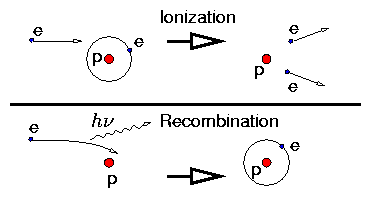Figure 1.1: Ionization and Recombination
Ionization has a threshold energy. Recombination has not but is much less probable.
Threshold is ionization energy (13.6eV, H) written χi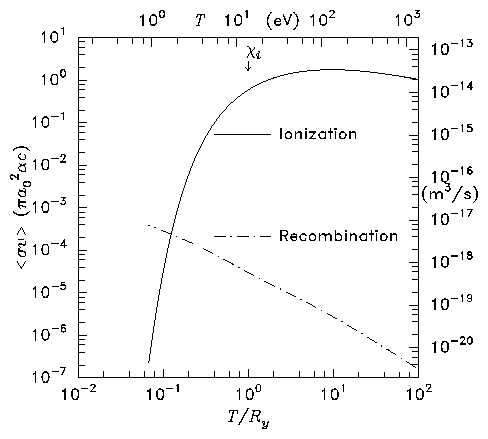Figure 1.2: Ionization and radiative recombination rate coefficients for atomic hydrogen
Integral over Maxwellian distribution gives rate coefficients (reaction rates). Because of the tail of the Maxwellian distribution, the ionization rate extends below T = χi. And in equilibrium, when
 nions nneutrals = < σi v > < σr v > ,
(1.1)
the percentage of ions is large ( ∼  100%) if electron temperature: Te >~χi/10. e.g. Hydrogen is ionized for Te >~1eV (11,600°K). At room temperature ionization is negligible.
For dissociation and ionization balance figure see e.g. Delcroix Plasma Physics Wiley (1965) figure 1A.5, page 25.

### 1.1.2  Plasmas are Quasi-Neutral

If a gas of electrons and ions (singly charged) has unequal numbers, there will be a net charge density, ρ.
 ρ = ne(−e) + ni(+e) = e (ni − ne)
(1.2)
This will give rise to an electric field via
 ∇ . E= ρ ϵ0 = e ϵ0 (ni − ne)
(1.3)

#### Example: Slab.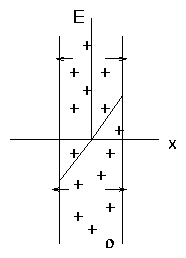Figure 1.3: Charged slab

 dE dx
 =
 ρ ϵ0
(1.4)
 Solution:       E
 =
 ρ x ϵ0
(1.5)
This results in a force on the charges tending to expel whichever species is in excess. That is, if ni > ne, the E field causes ni to decrease, ne to increase tending to reduce the charge.
This restoring force is enormous!
Example
Consider Te = 1eV, ne = 1019m−3 (a modest plasma; c.f. density of atmosphere nmolecules  ∼ 3 ×1025m−3). Suppose there is a small difference in ion and electron densities ∆n = (ni − ne)
 so    ρ = ∆n e
(1.6)
Then the force per unit volume at distance x is
 Fe = ρE = ρ2 x ϵ0 = (∆n e)2 x ϵ0
(1.7)
Take ∆n / ne = 1%  , x = 0.10 m.
 Fe = (1017 ×1.6 ×10−19)2 0.1 / 8.8 ×10−12 = 3 ×106 N.m−3
(1.8)
Compare with this the pressure force per unit volume  ∼ p/x with p  ∼ ne Te (+ ni Ti)
 Fp  ∼ 1019 ×1.6 ×10−19 / 0.1 = 16 Nm−3
(1.9)
Electrostatic force >> Kinetic Pressure Force.
This is one aspect of the fact that, because of being ionized, plasmas exhibit all sorts of collective behavior, different from neutral gases, mediated by the long distance electromagnetic forces E, B.
Another example (related) is that of longitudinal waves. In a normal gas, sound waves are propagated via the intermolecular action of collisions. In a plasma, waves can propagate when collisions are negligible because of the coulomb interaction of the particles.

## 1.2  Plasma Shielding

### 1.2.1  Elementary Derivation of the Boltzmann Distribution

Basic principle of Statistical Mechanics:
Thermal Equilibrium ↔ Most Probable State i.e. State with largest number of possible arrangements of micro-states.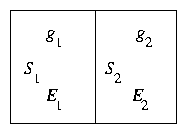Figure 1.4: Statistical Systems in Thermal Contact
Consider two weakly coupled systems S1, S2 with energies E1, E2. Let g1, g2 be the number of microscopic states which give rise to these energies, for each system. Then the total number of micro-states of the combined system assuming states are independent is
 g = g1 g2
(1.10)
If the total energy of combined system is fixed E1 + E2 = Et then this can be written as a function of E1:
 g
 =
 g1 (E1) g2 (Et − E1)
(1.11)
 and dg dE1
 =
 dg1 dE g2 − g1 dg2 dE .
(1.12)
The most probable state is that for which [dg/(dE1)] = 0 i.e.
 1 g1 dg1 dE = 1 g2 d g2 dE or d dE lng1 = d dE lng2
(1.13)
Thus, in equilibrium, states in thermal contact have equal values of [d/dE] lng.
One defines σ ≡ lng as the Entropy.
And [ [d/dE] lng ]−1 = T the Temperature.
Now suppose that we want to know the relative probability of 2 micro-states of system 1 in equilibrium. There are, in all, g1 of these states, for each specific E1 but we want to know how many states of the combined system correspond to a single microstate of S1.
Obviously that is just equal to the number of states of system 2. So, denoting the two values of the energies of S1 for the two microstates we are comparing by EA, EB the ratio of the number of combined system states for S1A and S1B is
 g2 (Et − EA) g2 (Et − EB) = exp[ σ(Et − EA) − σ(Et − EB) ]
(1.14)
Now we suppose that system S2 is large compared with S1 so that EA and EB represent very small changes in S2's energy, and we can Taylor expand the entropy of S2 (i.e. σ)
 g2 (Et − EA) g2 (Et − EA) ≅  exp ⎡⎣ − EA dσ dE + EB d σ dE ⎤⎦
(1.15)
Thus we have shown that the ratio of the probability of a system (S1) being in any two micro-states A, B is simply
 exp ⎡⎣ − (EA − EB) T ⎤⎦ ,
(1.16)
when in equilibrium with a (large) thermal "reservoir". This is the well-known "Boltzmann factor".
You may notice that Boltzmann's constant (kB) is absent from this formula. That is because of using natural thermodynamic units for entropy (dimensionless) and temperature (energy).
Boltzmann's constant is simply a conversion factor between the natural units of temperature (energy, e.g. Joules) and (e.g.) degrees Kelvin. Kelvins are based on °C which arbitrarily choose melting and boiling points of water and divide into 100 intervals. Temperature in Joules = kB× temperature in Kelvins.
Plasma physics is done almost always using energy units for temperature. Because Joules are very large, usually electron-volts (eV) are used.
 1 eV = 11600 K = 1.6 ×10−19 Joules.
(1.17)
One consequence of our Botzmann factor is that a gas of moving particles whose energy is 1/2 mv2 adopts the Maxwell-Boltzmann (Maxwellian) distribution of velocities ∝ exp[ − [(mv2)/2T] ].

### 1.2.2  Plasma Density in Electrostatic Potential

When there is a varying potential, ϕ, the densities of electrons (and ions) is affected by it.
If electrons are in thermal equilibrium, they will adopt a Boltzmann distribution of density
 ne ∝ exp( eϕ Te )   .
(1.18)
This is because each electron, regardless of velocity possesses a potential energy −eϕ.
Consequence is that (fig 1.5)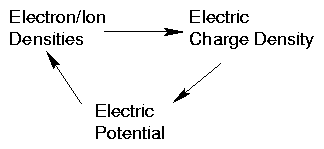Figure 1.5: Self-consistent loop of dependencies
a self-consistent loop of dependencies occurs.
This is one elementary example of the general principle of plasmas requiring a self-consistent solution of Maxwell's equations of electrodynamics plus the particle dynamics of the plasma.

### 1.2.3  Debye Shielding

A slightly different approach to discussing quasi-neutrality leads to the important quantity called the Debye Length.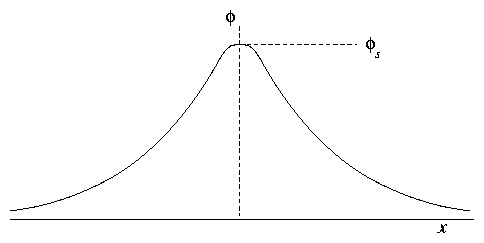Figure 1.6: Shielding of fields from a 1-D grid.
Suppose we put a plane grid into a plasma, held at a certain potential, ϕg.
Then, unlike the vacuum case, the perturbation to the potential falls off rather rapidly into the plasma. We can show this as follows. The important equations are:
 Poisson′s  Equation
 ∇2 ϕ = d2ϕ dx2 =  − e ϵ0 (ni − ne)
(1.19)
 Electron  Density
 ne = n∞ exp(e ϕ/Te) .
(1.20)
[This is a Boltzmann factor; it assumes that electrons are in thermal equilibrium. n is density far from the grid (where we take ϕ = 0).]
 Ion Density      ni = n∞  .
(1.21)
This approximation applies far from grid by quasineutrality; we just assume, for the sake of this illustrative calculation that ion density is not perturbed by ϕ-perturbation. That is not always the case.
Substitute:
 d2 ϕ d x2 = e n∞ ϵ0 ⎡⎣ exp ⎛⎝ e ϕ Te ⎞⎠ − 1 ⎤⎦ .
(1.22)
This is a nasty nonlinear equation, but far from the grid |e ϕ/ Te | << 1 so we can use a Taylor expression: exp[(e ϕ)/(Te)] ≅ 1 + [( e ϕ)/(Te)]. So
 d2 ϕ d x2 = e n∞ ϵ0 e Te ϕ = e2 n∞ ϵ0 Te ϕ
(1.23)
Solutions: ϕ = ϕ0 exp(− |x| / λD ) where
 λD ≡ ⎛⎝ ϵ0 Te e2 n∞ ⎞⎠ 1/2
(1.24)
This is called the Debye Length
Perturbations to the charge density and potential in a plasma tend to fall off with characteristic length λD.
In magnetic fusion plasmas λD is typically small. [e.g. ne = 1020 m−3 Te = 1keV    λD = 2 ×10−5m = 20 μm.] In space plasmas, which have far lower density, it can be much larger: hundreds of meters or more.
Usually we include as part of the definition of a plasma that λD << the size of plasma. This ensures that collective effects, quasi-neutrality etc. are important. Otherwise they probably aren't.

### 1.2.4  Plasma-Solid Boundaries  (Elementary)

When a plasma is in contact with a solid, the solid acts as a "sink" draining away the plasma. Recombination of electrons and ions occur at surface. Then:
1. Plasma is normally charged positively with respect to the solid.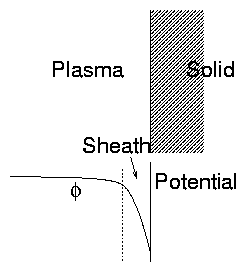Figure 1.7: Plasma-Solid interface: Sheath
2. There is a relatively thin region called the "sheath", at the boundary of the plasma, where the main potential variation occurs.
Reason for potential drop:
Different velocities of electrons and ions.
If there were no potential variation (E= 0) the electrons and ions would hit the surface at the random rate
 1 4 n - v per unit area
(1.25)
[This equation comes from elementary gas-kinetic theory. See problems if not familiar.]
The mean speed v = √{ [8 T/(πm)]}  ∼ √{ T/m}.
Because of mass difference electrons move  ∼ √{ [(mi)/(me)]} faster and hence would drain out of plasma faster. Hence, plasma charges up enough that an electric field opposes electron escape and reduces total electric current to zero.
Estimate of potential:
Ion escape flux            1/4 n′ivi
Electron escape flux    1/4 n′e vi
Prime denotes values at solid surface.
Boltzmann factor applied to electrons:
 n′e = n∞ exp[ e ϕs / Te ]
(1.26)
where ϕs is solid potential relative to distant (∞) plasma.
Since ions are being dragged out by potential assume n′i  ∼ n (Zi = 1). [This is only approximately correct.]
Hence total current density out of plasma is
 j
 =
 qi 1 4 n′i - v i + qe 1 4 n′e - v e
(1.27)
 =
 e n∞ 4 { - v i − exp ⎡⎣ e ϕs Te ⎤⎦ - v e }
(1.28)
This must be zero so
 ϕs
 =
Te

e
ln|
 - v i

 - v e
| = Te

e
1

2
ln
Ti

Te
me

mi

(1.29)
 =
 Te e 1 2 ln ⎛⎝ me mi ⎞⎠ [if Te = Ti.]
(1.30)
For hydrogen [( mi)/(me)] = 1800 so 1/2 ln[(me)/(mi)] = − 3.75.
The potential of the surface relative to plasma is approximately −4 [(Te)/e].
[Note [(Te)/e] is just the electron temperature in electron-volts expressed as a voltage.]

### 1.2.5  Thickness of the sheath

Crude estimates of sheath thickness can be obtained by assuming that ion density is uniform. Then equation of potential is, as before,
 d2 ϕ d x2 = e n∞ ϵ0 ⎡⎣ exp ⎛⎝ eϕ Te ⎞⎠ − 1 ⎤⎦
(1.31)
We know the rough scale-length of solutions of this equation is
 λD = ⎛⎝ ϵ0Te e2 n∞ ⎞⎠ 1/2 the Debye Length.
(1.32)
Actually our previous solution was valid only for |eϕ/Te| << 1 which is no longer valid.
When −eϕ/Te > 1 (as will be the case in the sheath). We can practically ignore the electron density, in which case the solution will continue only quadratically. One might expect, therefore, that the sheath thickness is roughly given by an electric potential gradient
 − T e 1 λD
(1.33)
extending sufficient distance to reach ϕS = − 4 [( Te)/e] i.e.
distance x  ∼ 4 λD
This is correct for the typical sheath thickness but not at all rigorous.

## 1.3  The `Plasma Parameter'

Notice that in our development of Debye shielding we used nee as the charge density and supposed that it could be taken as smooth and continuous. However if the density were so low that there were less than approximately one electron in the Debye shielding region this approach would not be valid. Actually we have to address this problem in 3-d by defining the `Plasma Parameter', ND, as
ND = Number of particles in the `Debye Sphere'.

 = n . 4 3 πλ3D ⎛⎝ ∝ T3/2 n1/2 ⎞⎠ .
(1.34)
If ND <~1 then the individual particles cannot be treated as a smooth continuum. It will be seen later that this means that collisions dominate the behaviour: i.e. short range correlation is just as important as the long range collective effects.
Often, therefore we add a further qualification of plasma:
 ND >> 1    (Collective effects dominate over collisions)
(1.35)

## 1.4  Summary

Plasma is an ionized gas in which collective effects dominate over collisions.
 [ λD << size    ,    ND >> 1   .]
(1.36)

## 1.5  Occurrence of Plasmas

Gas Discharges:    Fluorescent Lights, Spark gaps, arcs, welding, lighting
Controlled Fusion
Ionosphere:         Ionized belt surrounding earth
Interplanetary Medium:    Magnetospheres of planets and starts. Solar Wind.
Ion Propulsion:         Advanced space drives, etc.
& Space Technology      Interaction of Spacecraft with environment
Gas Lasers:           Plasma discharge pumped lasers: CO2, He, Ne, HCN.
Materials Processing:    Surface treatment for hardening. Crystal Growing.
Semiconductor Processing:  Ion beam doping, plasma etching & sputtering.
Solid State Plasmas:   Behavior of semiconductors.
For a figure locating different types of plasma in the plane of density versus temperature see for example Goldston and Rutherford Introduction to Plasma Physics IOP Publishing, 1995, figure 1.3 page 9. Another is at http://www.plasmas.org/basics.htm

## 1.6  Different Descriptions of Plasma

1. Single Particle Approach. (Incomplete in itself). Eq. of Motion.
2. Kinetic Theory. Boltzmann Equation.
 ⎡⎣ ∂ ∂t + v. ∂ ∂x + a . ∂ ∂v ⎤⎦ f  = ∂f ∂t ⎞⎠ col.
(1.37)
3. Fluid Description. Moments, Velocity, Pressure, Currents, etc.
Uses of these.
Single Particle Solutions → Orbits
→ Kinetic Theory Solutions → Transport Coefs.
→ Fluid Theory → Macroscopic Description
All descriptions should be consistent. Sometimes they are different ways of looking at the same thing.

### 1.6.1  Equations of Plasma Physics

 ∇. E
 =
 ρ ϵ0
 ∇. B
 =
 0
 ∇∧E
 =
 − ∂B ∂t
 ∇∧B
 =
 μ0 j + 1 c2 ∂E ∂t
 F = q ( E+ v∧B)
(1.38)

### 1.6.2  Self Consistency

In solving plasma problems one usually has a `circular' system:
The problem is solved only when we have a model in which all parts are self consistent. We need a `bootstrap' procedure.
Generally we have to do it in stages:
• Calculate Plasma Response (to given E,B)
• Get currents & charge densities
• Calculate E & B for j, p.
Then put it all together. This will become clearer by example as we develop the subject.